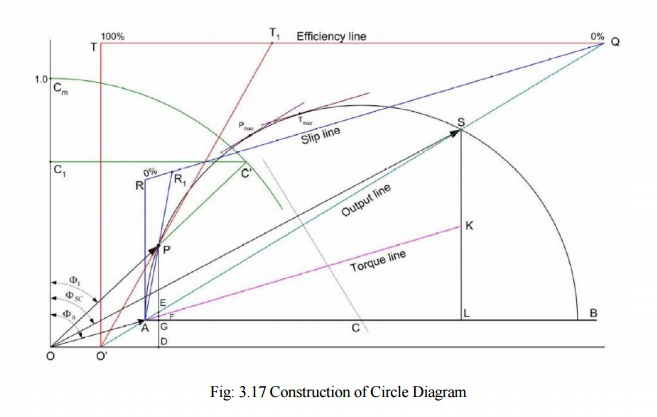Home | | Basic Electrical and Instrumentation Engineering | | Electrical Machines II | Construction of Circle Diagram - Induction Motor

# Construction of Circle Diagram - Induction MotorConduct No load test and blocked rotor test on the induction motor and find out the per phase values of no load current I0, short circuit current ISC and the corresponding phase angles Ф0 and ФSC.

Construction of Circle Diagram

Conduct No load test and blocked rotor test on the induction motor and find out the per phase values of no load current I0, short circuit current ISC and the corresponding phase angles Ф0 and ФSC. Also find short circuit current ISN corresponding to normal supply voltage. With this data, the circle diagram can be drawn as follows see Fig: 3.17.

1. With suitable scale, draw vector OA with length corresponding to I0 at an angle      Ф0 from the vertical axis. Draw a horizontal line AB.

2. Draw OS equal to ISN at an angle ФSC and join AS.

3.                                  Draw the perpendicular bisector to AS to meet the horizontal line AB at C.

4.                                  With C as centre, draw a portion of circle passing through A and S. This forms the circle diagram which is the locus of the input current.

5.                                  From point S, draw a vertical line SL to meet the line AB.

6.                                  Divide SL at point K so that SK : KL = rotor resistance : stator resistance.

7.                                  For a given operating point P, draw a vertical line PEFGD as shown. then PE = output power, EF = rotor copper loss, FG = stator copper loss, GD = constant loss (iron loss + mechanical loss)

8.                                  To find the operating points corresponding to maximum power and maximum torque, draw tangents to the circle diagram parallel to the output line and torque line respectively. The points at which these tangents touch the circle are respectively the maximum power point and maximum torque point.## Efficiency line

1.           The output line AS is extended backwards to meet the X-axis at O′.

2.           From any convenient point on the extended output line, draw a horizontal line QT so as to meet the vertical from O′. Divide the line QT into 100 equal parts.

3.           To find the efficiency corresponding to any operating point P, draw a line from O′ to the efficiency line through P to meet the efficiency line at T1. Now QT1 is the efficiency.

## Slip Line

1.           Draw line QR parallel to the torque line, meeting the vertical through A at R. Divide RQ into 100 equal parts.

2.           To find the slip corresponding to any operating point P, draw a line from A to the slip line through P to meet the slip line at R1. Now RR1 is the slip

## Power Factor Curve

1.           Draw a quadrant of a circle with O as centre and any convenient radius. Divide OCm into 100 equal parts.

2.           To find power factor corresponding to P, extend the line OP to meet the power factor curve at C′. Draw a horizontal line C′C1 to meet the vertical axis at C1. Now OC1 represents power factor.

Study Material, Lecturing Notes, Assignment, Reference, Wiki description explanation, brief detail

Related Topics

Copyright © 2018-2021 BrainKart.com; All Rights Reserved. (BS) Developed by Therithal info, Chennai.